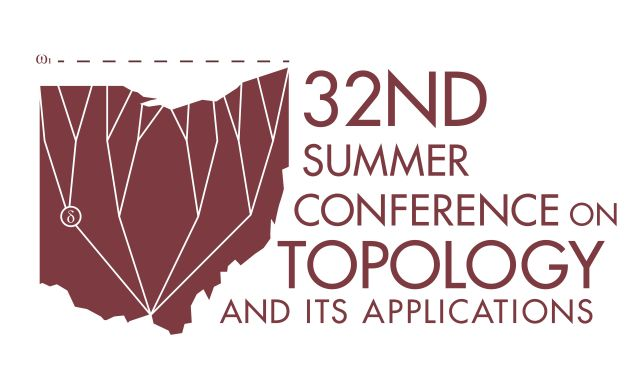#### Document Type

Topology + Foundations

6-2017

#### Publication Source

32nd Summer Conference on Topology and Its Applications

#### Abstract

In this paper an exact homology functor from the category MorC of continuous maps of compact Hausdorff spaces to the category LES of long exact sequences of abelian groups is defined (cf. , , ). This functor is an extension of the Hu homology theory, which is uniquely defined on the category of continuous maps of finite CW complexes and is constructed without the relative homology groups . To define the given homology functor we use the Chogoshvili construction of projective homology theory , . For each continuous map f:X → Y of compact spaces, using the notion of the partition of spaces ,  and approximations of continuous maps , , , V. Baladze defined the inverse system f = { fλ, pλλ', Λ }, where Λ is the directed system of pairs λ = (α, β) of partitions, where α is refined in f-1(β) and fλ: Xα → Yβ is the simplicial map of nerves Xα and Yβ of closed coverings defined by partitions α and β. Using this system he has defined and studied the inverse system of chain complexes C* (f) = { C*(fλ), pλλ'#, Λ } and the projective and spectral homology groups:

- H * (f) ≡ H*(\varprojlim { C*(fλ), pλλ'#, Λ }) ^ H * (f) ≡ \varprojlim ({ H*(fλ), pλλ'#, Λ }).

A. Beridze has shown that there exists a Milnor short exact sequence (cf. , , ), which connects the defined homology groups:

0 → \varprojlim1 Hn+1(fλ) → - H * (f) → ^ H * (f) → 0.

Using this property, he has shown that the homology groups H̅*(f) satisfy the universal coefficient formula:

0 → Ext ( ^ H n+1 (f);G) → - H * (f) → Hom( ^ H n (f);G) → 0.

Consequently, using the methods developed in ,  and , we have shown that the constructed functor is unique on the category MorC.

This document is available for download with the permission of the presenting author and the organizers of the conference. Permission documentation is on file.

Technological limitations may prevent some mathematical symbols and functions from displaying correctly in this record’s metadata fields. Please refer to the attached PDF for the correct display.

COinS